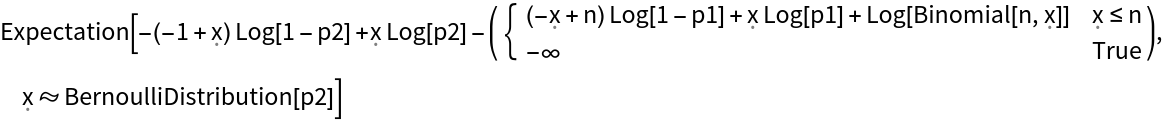#Function Repository Resource:

# KullbackLeiblerDivergence

Calculate the Kullback–Leibler divergence between two distributions

Contributed by: Sjoerd Smit
 ResourceFunction["KullbackLeiblerDivergence"][P,Q] computes DKL(P∥Q), the Kullback–Leibler divergence from distribution Q to distribution P.

## Details and Options

The Kullback–Leibler divergence (DKL) is an asymmetric measure of dissimilarity between two probability distributions P and Q. If it can be computed, it will always be a number ≥0 (with equality if and only if the two distributions are the same almost everywhere). In a Bayesian setting, it represents the information gained when updating a prior distribution Q to posterior distribution P. Conversly, it can be seen as the information lost when Q is used to approximate P. Because DKL is assymetric, DKL(PQ) is generally different from DKL(QP).
P and Q must both satistfy DistributionParameterQ.
The support of P must be contained in the support of Q. In other words: the random variable P may never take values that Q can't take. Intuitively, this is because any value excluded by the prior will automatically also be excluded by the posterior. If the supports do not satisfy this constraint, ResourceFunction["KullbackLeiblerDivergence"] will raise a message and return Undefined.
ResourceFunction["KullbackLeiblerDivergence"] works for multivariate distributions.
ResourceFunction["KullbackLeiblerDivergence"] has a Method option that allows the user to specify if the computation should be done with Expectation (default) or NExpectation. Sub-options for these functions can also be provided. For example, to pass suboptions into Expectation you can use Method {Expectation,opt1val1,}.

## Examples

### Basic Examples

Calculate the KullbackLeiblerDivergence between two NormalDistributions:

 In:=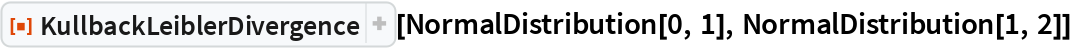Out=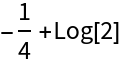KullbackLeiblerDivergence is not symmetric:

 In:=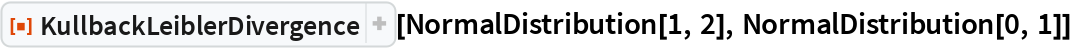Out=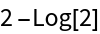In:=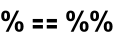Out=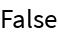KullbackLeiblerDivergence works with symbolic distributions:

 In:=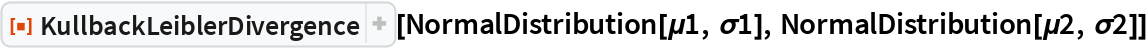Out=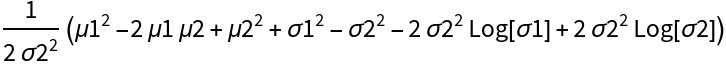The distributions do not have to be from the same family:

 In:=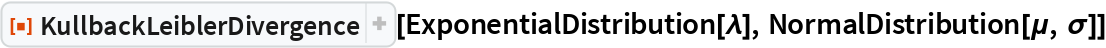Out=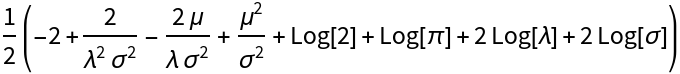It also works with discrete distributions:

 In:=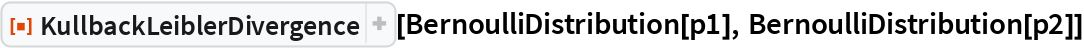Out=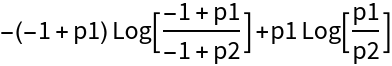In:=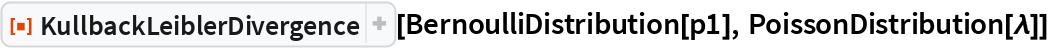Out=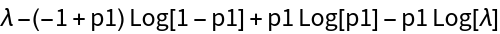Use a custom defined ProbabilityDistribution:

 In:=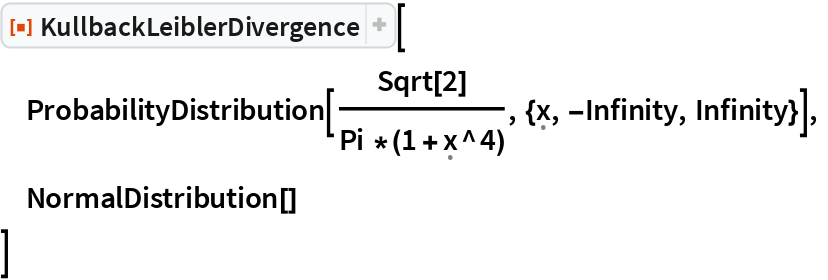Out=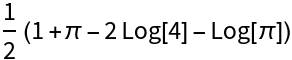### Scope

KullbackLeiblerDivergence works for multivariate distributions:

 In:=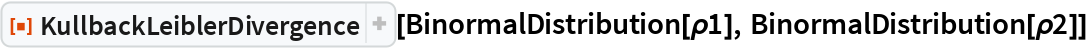Out=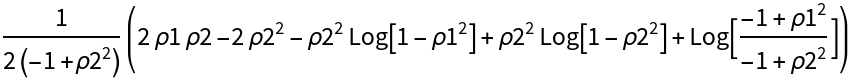KullbackLeiblerDivergence also works with EmpiricalDistribution:

 In:=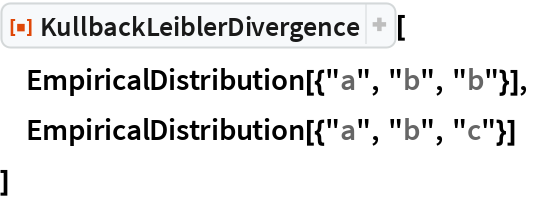Out=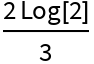In:=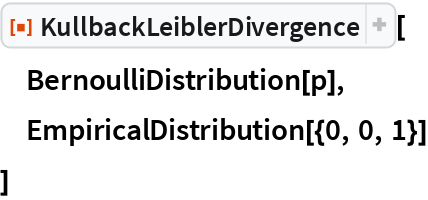Out=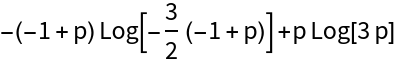### Options

#### Method

Symbolic evaluation of the divergence is unfeasible for some distributions:

 In:=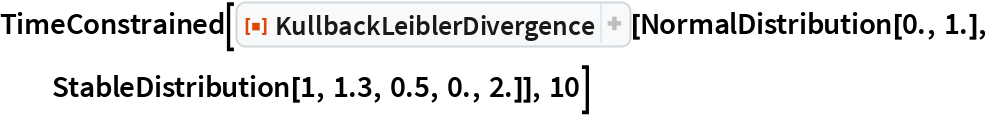Out=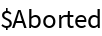In:=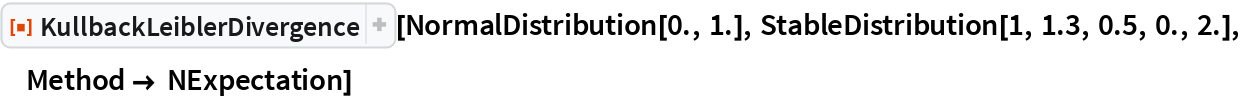Out=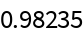Supply extra options to NExpectation:

 In:=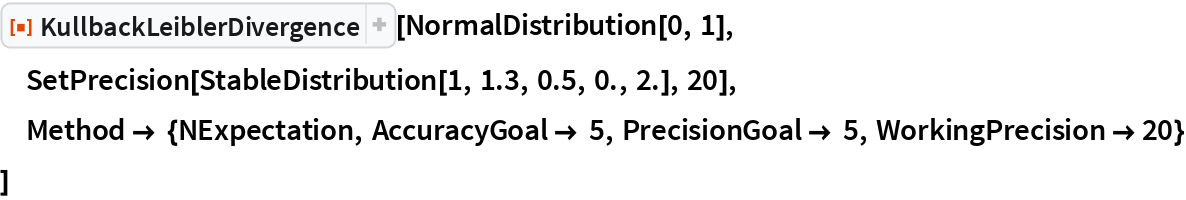Out=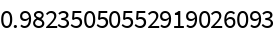NExpectation is designed to work with distributions with numeric parameters:

 In:=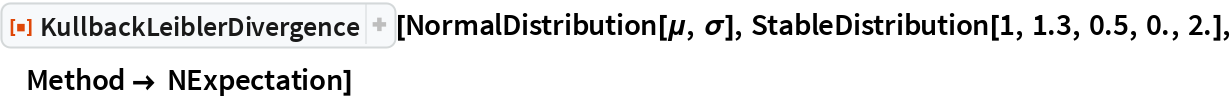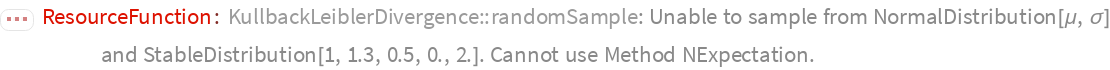Out=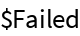#### Assumptions

Without Assumptions, a message will be raised and conditions are generated in the result:

 In:=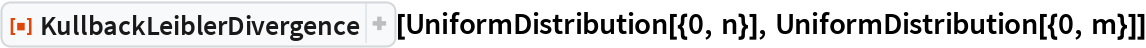Out=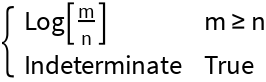With assumptions specified, a result valid under those conditions is returned:

 In:=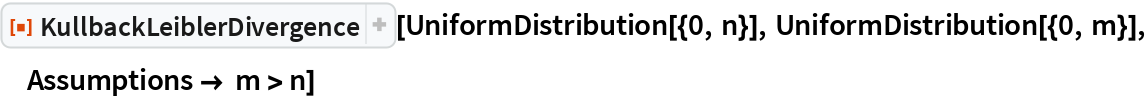Out=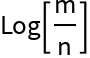### Applications

If X and Y are two random variables with a joint distribution 𝒟, then the mutual information between them is defined as the Kullback–Leibler divergence from the product distribution of the marginals to 𝒟.

As an example, calculate the mutual information of the components of a BinormalDistribution:

 In:=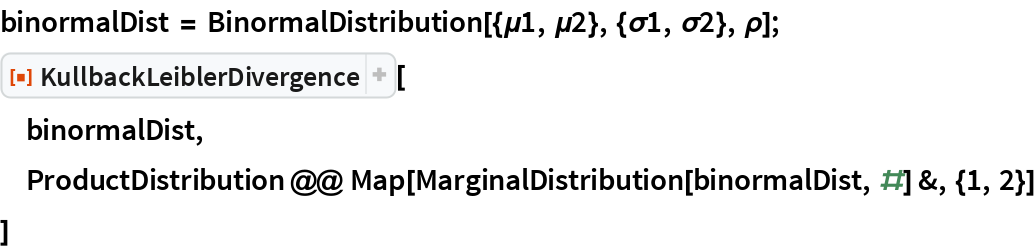Out=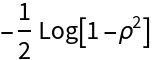The Kullback–Leibler divergence can be used to fit distributions to data and also provides a measure of the quality of the fit in a way very similar to maximum likelihood estimation. First generate some samples from a discrete distribution:

 In:=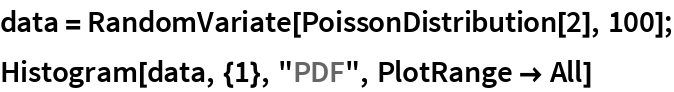Out=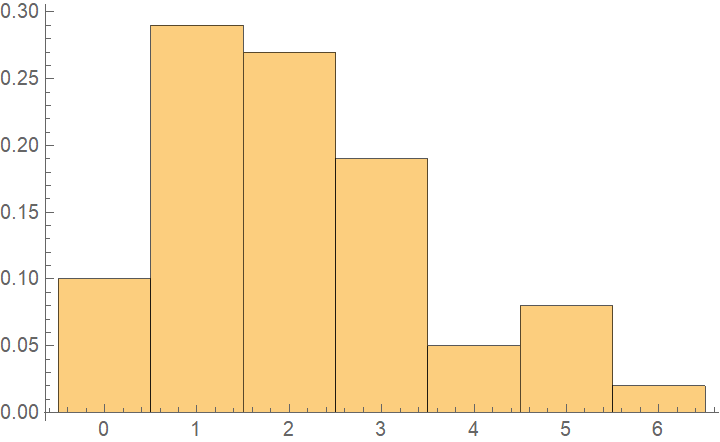Calculate the divergence from the EmpiricalDistribution to a symbolic target distribution:

 In:=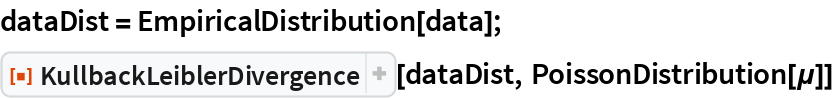Out=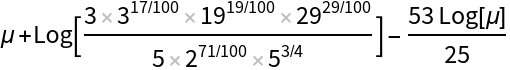Minimize with respect to μ:

 In:=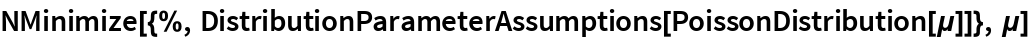Out=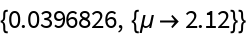Try a different distribution to compare:

 In:=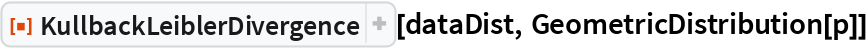Out=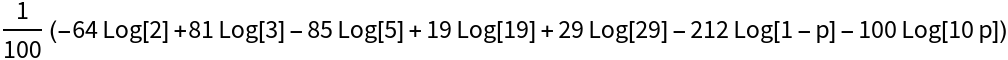The minimized divergence is larger, indicating this distribution is a worse approximation to the data:

 In:=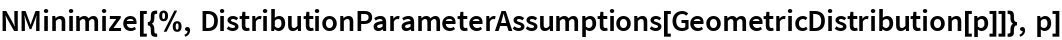Out=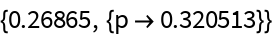Note that the reverse divergences are not defined because the supports of PoissonDistribution and GeometricDistribution are infinite:

 In:=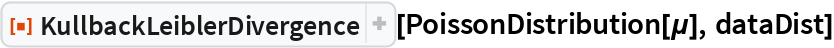Out=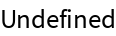Also note that this does not work for continuous data because the support of EmpiricalDistribution is discrete:

 In:=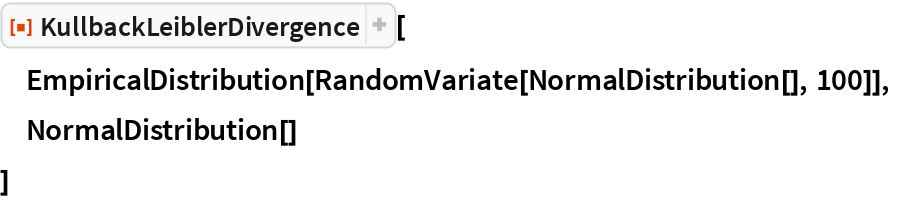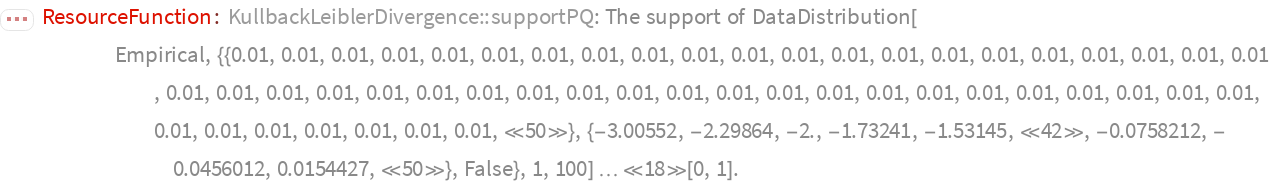Out=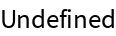Use a KernelMixtureDistribution instead for continuous data:

 In:=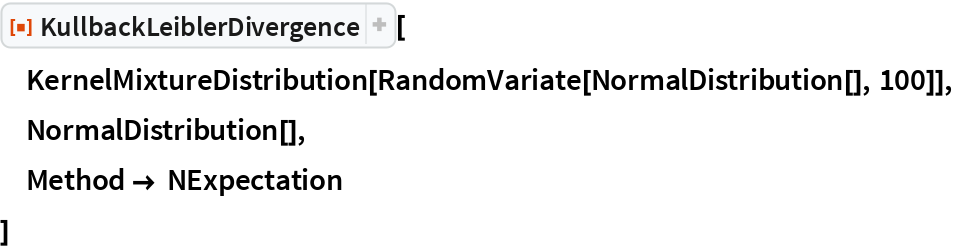Out=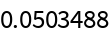### Properties and Relations

The divergence from a distribution to itself is zero:

 In:=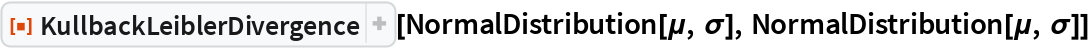Out=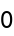### Possible Issues

The dimensions of the distributions have to match:

 In:=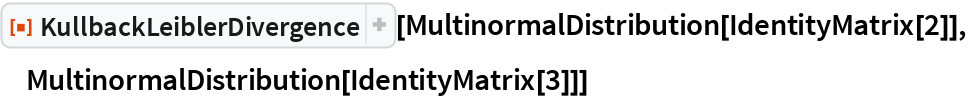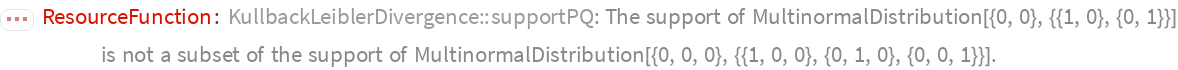Out=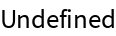Matrix distributions are currently not supported:

 In:=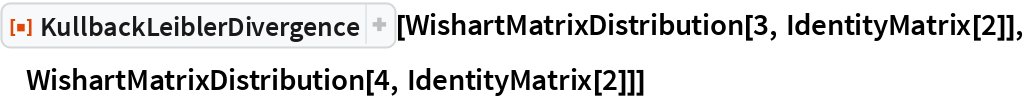Out=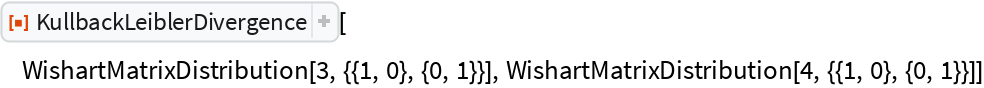The divergence is Undefined if the first distribution has a wider support than the second:

 In:=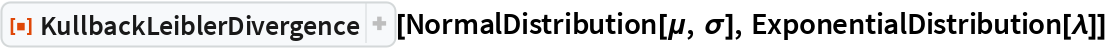Out=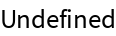In:=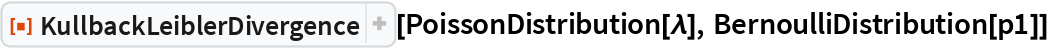Out=KullbackLeiblerDivergence is undefined between discrete and continuous distributions:

 In:=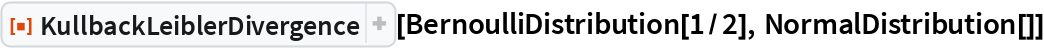Out=For some symbolic distributions the expectation cannot be evaluated:

 In:=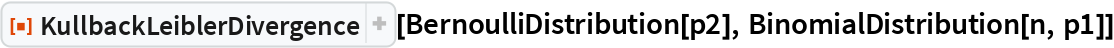Out=